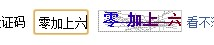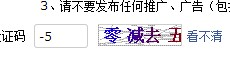# 【四圣龙神录的编程教室】第18章、给自机的子弹加上碰撞检测吧

＞if(cshot[i].cnt>0){

————————————————————————————————————————————————————————————————————————

---- out.cpp を変更 ----

#include "../include/GV.h"

#define ENEMY_RANGE_MAX 4
#define CSHOT_RANGE_MAX 2

//敌机的碰撞检测范围
int enemy_range[ENEMY_RANGE_MAX]={16,30,16,50};
//自机的子弹的碰撞检测范围
int cshot_range[CSHOT_RANGE_MAX]={6,};

//判断是否发生碰撞
int out_judge_cshot(int i,int s){
int j;
if(cshot[i].cnt>0){//每一帧都对子弹的轨道进行计算
double x=cshot[i].x-enemy[s].x;//敌机和自机子弹的距离
double y=cshot[i].y-enemy[s].y;
//超界的判断
if(cshot[i].knd>=CSHOT_RANGE_MAX || enemy[s].knd>=ENEMY_RANGE_MAX)
printfDx("out_judge_cshot内オーバーフロー");
//敌机的判定和自己子弹判定的加起来的范围
double r=cshot_range[cshot[i].knd]+enemy_range[enemy[s].knd];
//如果需要中间计算
if(cshot[i].spd>r){
//保存下前一帧所在的位置
double pre_x=cshot[i].x+cos(cshot[i].angle+PI)*cshot[i].spd;
double pre_y=cshot[i].y+sin(cshot[i].angle+PI)*cshot[i].spd;
double px,py;
for(j=0;j<cshot[i].spd/r;j++){//前进的部分除以碰撞判定的部分，循环这么多次
px=pre_x-enemy[s].x;
py=pre_y-enemy[s].y;
if(px*px+py*py<r*r)
return 1;
pre_x+=cos(cshot[i].angle)*r;
pre_y+=sin(cshot[i].angle)*r;
}
}
if(x*x+y*y<r*r)//检测在碰撞内的话
return 1;//碰撞
}
return 0;
}

//判断敌机是否死亡
void enemy_death_judge(int s){
int i;
se_flag=1;//敌机被打中的音效
if(enemy[s].hp<0){//敌机的血量小于0的话
enemy[s].flag=0;//让敌机消失
se_flag=1;//敌机的 BIU 的音效
for(i=0;i<SHOT_MAX;i++){//遍历所有的弹幕
if(shot[i].flag!=0){//如果有注册了的弹幕数据
if(s==shot[i].num){//如果这个弹幕是这个敌机发出来的
shot[i].flag=2;//设置标记，让这个弹幕不再持续了
break;
}
}
}
}
}

//碰撞检测，主函数
void out_main(){
int i,s;
for(i=0;i<CSHOT_MAX;i++){//自机的子弹总数
if(cshot[i].flag>0){
for(s=0;s<ENEMY_MAX;s++){//敌机总数
if(enemy[s].flag>0){
if(out_judge_cshot(i,s)){//自机子弹和敌机碰撞的话
cshot[i].flag=0;//让那个自机的子弹消失
enemy[s].hp-=cshot[i].power;//根据子弹的威力，减去对应的敌机HP
enemy_death_judge(s);//判断敌机是否死亡
}
}
}
}
}
}

——————————————————————————————————————————————————————————————————————————

——————————————————————————————————————————————————————————————————————————

---- main.cpp 做如下改动 ----

case 100://通常处理
calc_ch();   //自机的处理
ch_move();   //自机的移动操作
cshot_main();//自机的射击主函数
enemy_main();//敌机的处理的主函数
shot_main(); //射击的主函数
out_main();  //碰撞检测
graph_main();//绘画的主函数
stage_count++;
break;

---- function.h 里做如下改动 ----

//out.cpp
GLOBAL void out_main();

ChangeVolumeSoundMem( 50, sound_se ) ;//设定各个素材的回放音量
ChangeVolumeSoundMem(128, sound_se ) ;
ChangeVolumeSoundMem(128, sound_se ) ;
ChangeVolumeSoundMem( 80, sound_se ) ;

---- music.cpp 的 music_play改成如下这样 ----

void music_play(){
int i;
for(i=0;i<SE_MAX;i++){
if(se_flag[i]==1){
if(CheckSoundMem(sound_se[i])!=0){
if(i==8)continue;
StopSoundMem(sound_se[i]);
}
PlaySoundMem(sound_se[i],DX_PLAYTYPE_BACK);
}
}
}

——————————————————————————————————————————————————————————————————————————

if(i==8)continue;

Excel 文件作适当的变更。

http://dixq.net/rp/swf/18.swf

＞if(cshot[i].cnt>0){

————————————————————————————————————————————————————————————————————————

---- out.cpp を変更 ----

#include "../include/GV.h"

#define ENEMY_RANGE_MAX 4
#define CSHOT_RANGE_MAX 2

//敌机的碰撞检测范围
int enemy_range[ENEMY_RANGE_MAX]={16,30,16,50};
//自机的子弹的碰撞检测范围
int cshot_range[CSHOT_RANGE_MAX]={6,};

//判断是否发生碰撞
int out_judge_cshot(int i,int s){
int j;
if(cshot[i].cnt>0){//每一帧都对子弹的轨道进行计算
double x=cshot[i].x-enemy[s].x;//敌机和自机子弹的距离
double y=cshot[i].y-enemy[s].y;
//超界的判断
if(cshot[i].knd>=CSHOT_RANGE_MAX || enemy[s].knd>=ENEMY_RANGE_MAX)
printfDx("out_judge_cshot内オーバーフロー");
//敌机的判定和自己子弹判定的加起来的范围
double r=cshot_range[cshot[i].knd]+enemy_range[enemy[s].knd];
//如果需要中间计算
if(cshot[i].spd>r){
//保存下前一帧所在的位置
double pre_x=cshot[i].x+cos(cshot[i].angle+PI)*cshot[i].spd;
double pre_y=cshot[i].y+sin(cshot[i].angle+PI)*cshot[i].spd;
double px,py;
for(j=0;j<cshot[i].spd/r;j++){//前进的部分除以碰撞判定的部分，循环这么多次
px=pre_x-enemy[s].x;
py=pre_y-enemy[s].y;
if(px*px+py*py<r*r)
return 1;
pre_x+=cos(cshot[i].angle)*r;
pre_y+=sin(cshot[i].angle)*r;
}
}
if(x*x+y*y<r*r)//检测在碰撞内的话
return 1;//碰撞
}
return 0;
}

//判断敌机是否死亡
void enemy_death_judge(int s){
int i;
se_flag=1;//敌机被打中的音效
if(enemy[s].hp<0){//敌机的血量小于0的话
enemy[s].flag=0;//让敌机消失
se_flag=1;//敌机的 BIU 的音效
for(i=0;i<SHOT_MAX;i++){//遍历所有的弹幕
if(shot[i].flag!=0){//如果有注册了的弹幕数据
if(s==shot[i].num){//如果这个弹幕是这个敌机发出来的
shot[i].flag=2;//设置标记，让这个弹幕不再持续了
break;
}
}
}
}
}

//碰撞检测，主函数
void out_main(){
int i,s;
for(i=0;i<CSHOT_MAX;i++){//自机的子弹总数
if(cshot[i].flag>0){
for(s=0;s<ENEMY_MAX;s++){//敌机总数
if(enemy[s].flag>0){
if(out_judge_cshot(i,s)){//自机子弹和敌机碰撞的话
cshot[i].flag=0;//让那个自机的子弹消失
enemy[s].hp-=cshot[i].power;//根据子弹的威力，减去对应的敌机HP
enemy_death_judge(s);//判断敌机是否死亡
}
}
}
}
}
}

——————————————————————————————————————————————————————————————————————————

——————————————————————————————————————————————————————————————————————————

---- main.cpp 做如下改动 ----

case 100://通常处理
calc_ch();   //自机的处理
ch_move();   //自机的移动操作
cshot_main();//自机的射击主函数
enemy_main();//敌机的处理的主函数
shot_main(); //射击的主函数
out_main();  //碰撞检测
graph_main();//绘画的主函数
stage_count++;
break;

---- function.h 里做如下改动 ----

//out.cpp
GLOBAL void out_main();

ChangeVolumeSoundMem( 50, sound_se ) ;//设定各个素材的回放音量
ChangeVolumeSoundMem(128, sound_se ) ;
ChangeVolumeSoundMem(128, sound_se ) ;
ChangeVolumeSoundMem( 80, sound_se ) ;

---- music.cpp 的 music_play改成如下这样 ----

void music_play(){
int i;
for(i=0;i<SE_MAX;i++){
if(se_flag[i]==1){
if(CheckSoundMem(sound_se[i])!=0){
if(i==8)continue;
StopSoundMem(sound_se[i]);
}
PlaySoundMem(sound_se[i],DX_PLAYTYPE_BACK);
}
}
}

——————————————————————————————————————————————————————————————————————————

if(i==8)continue;

Excel 文件作适当的变更。

http://dixq.net/rp/swf/18.swf

http://blog.csdn.net/tidus5#### 软件测试入门、SQL、性能测试、测试管理工具

03-0207-1021万+

05-01

09-28

12-13

#### Python可以这样学（第一季：Python内功修炼）

02-23

03-0120万+

#### Vue.js 2.0之全家桶系列视频课程

04-21©️2020 CSDN 皮肤主题: 大白 设计师: CSDN官方博客点击重新获取扫码支付1.余额是钱包充值的虚拟货币，按照1:1的比例进行支付金额的抵扣。
2.余额无法直接购买下载，可以购买VIP、C币套餐、付费专栏及课程。余额充值